>

## NCERT Solutions for Class 11 Maths Chapter 10 Straight Lines Exercise 10.2

Chapter 10 Straight Lines Exercise 10.2 Class 11 Maths NCERT Solutions is prepared by Studyrankers experts that will be useful guide in the journey of preparation of exams. You can improve your problem solving skills just by practicing the questions. NCERT Solutions for Class 11 Maths prepared by Studyrankers are updated as per the latest CBSE syllabus.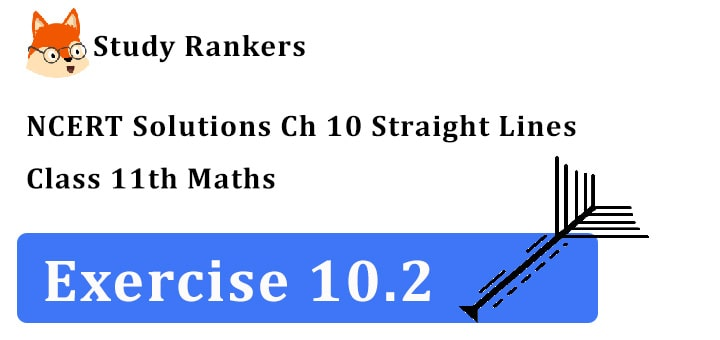1. Find the equation of the line, which satisfy the given condition. Write the equations for x and y-axis.

Equation of the x-axis is y = 0
Equation of the y-axis is x = 0

2. Find the equation of the line, which satisfy the given condition. Passing through (– 4, 3) with slope 1/2.

Equation of a line passing through (x, y) with slope m is (y – y 1) = m(x – x 1)
Here x 1 = – 4, y = 3, m = 1/2
∴ Equation of the line required is
(y – 3) = 1/2 (x + 4)
or 2y – 6 = x + 4 or x – 2y + 10 = 0

3. Find the equation of the line passing through (0, 0) with slope m.

The line passes through (0, 0) and its slope = m
Equation of the line is
y – y 1 = m(x – x 1)
=> y – 0 = m(x – 0)
=> y = mx

4. Find the equation of the line passing through (2, 2√3) and inclined with the x-axis at an angle of 75°.

We have x 1 = 2, y 1 = 2√3
m = tan 75°= tan (45° + 30°)
= (tan 45° + tan 30°)/(1 – tan 45° tan 30°) = (1 + 1/√3)/(1 – 1/√3) = (√3 + 1)/( √3 – 1)
Equation of the required line is,
y – 2√3 = (√3 + 1)/( √3 – 1) (x – 2)
=> (√3 – 1)y – 2√3(√3 – 1)
= (√3 + 1)x – 2(√3 + 1)
=> (√3 + 1)x – (√3 – 1)y
= -6 + 2√3 + 2√3 + 2
= 4√3 – 4 = 4(3√ – 1)
Then, equation of required line is
(√3 + 1)x – (√3 – 1)y = 4(√3 – 1)

5. Find the equation of the line intersecting the x-axis at a distance of 3 units to the left of origin with slope – 2.

The line passes through (– 3, 0) and has slope – 2.
Now, equation of the line is point-slope form is
y – y 1 = m(x – x 1)
y – 0 = –2(x + 3)
y = – 2x – 6
2x + y + 6 = 0
which is the required equation.

6. Find the equation of the line intersecting the y-axis at a distance of 2 units above the origin and making an angle of 30° with positive direction of the x-axis.

The line PQ intersects Y-axis at (0, 2)
Slope of PQ = tan 30° 1/√3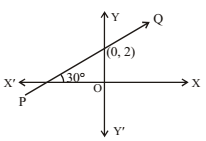Equation of PQ is y – 2 = 1/√3 (x – 0)
=> √3y – 2√3 = x => x –√3y + 2√3 = 0

7. Find the equation of the line passing through the points (–1, 1) and (2, –4).

Let the line passes through the points A(–1, 1) and B(2, –4).
Equation of the line passing through (x 1, y 1) and (x 2, y 2) is y – y1 = (y2 – y1)/(x2 – x1) (x – x1)
We have x 1 = –1, y 1 = 1, x 2 = 2, y 2 = – 4
∴ Equation of AB is y -1 = (-4 -1)/(2 + 1) (x + 1)
=> y – 1 = -5/3 (x + 1) => 3(y – 1) = –5(x + 1)
=> 3y – 3 = –5x – 5 => 5x + 3y – 3 + 5 = 0
=> 5x + 3y + 2 = 0

8. Find the equation of the line perpendicular distance from the origin is 5 units and the angle made by the perpendicular with the positive x-axis is 30°.

If a line is at a distance p from the origin and perpendicular make an angle ω with positive direction of x-axis then its equation is
xcosω + ysinω = p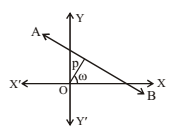We have p = 5, ω= 30°
∴ Equation of the required line is,
xcos 30° + ysin 30° = p
√3/2 x + 1/2 y = 5 => √3x + y = 10

9. The vertices of △PQR are P(2, 1), Q(–2, 3) and R(4, 5). Find equation of the median through the vertex R.

The middle point of PQ is S = ((2 – 2)/2 , (1 + 3)/2) or (0, 2)
∴ Equation of the median RS where R is (4, 5) and S is the point (0, 2) isy-5 = (2 – 5)/(0 – 4) . (x – 4)
or y – 5 = -3/-4  (x – 4)
or 4(y – 5) = 3(x – 4)
or 4y – 20 = 3x - 12
i.e. 3x – 4y = –8
∴ Equation of median RS is 3x – 4y + 8 = 0

10. Find the equation of the line passing through (–3, 5) and perpendicular to the line through the points (2, 5) and (–3, 6).

Slope of the line joining A(2, 5) and B(–3, 6)
= (6 – 5)/(-3 -2) = 1/-5
∴ m = slope of any line perpendicular to AB = 5
The equation of the line passing through (–3, 5) and perpendicular to AB is
y – 5 = 5(x + 3) => 5x – y + 20 = 0

11. A line perpendicular to the line segment joining the points (1, 0) and (2, 3) divides it in the ratio 1: n. Find the equation of the line.

Slope of the line joining the points A(1, 0) and B(2, 3) = ( 3 – 0)/(2 – 1) = 3/1 = 3
∴ Slope of the CD perpendicular to
AB= - (1/3)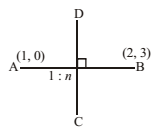[∵ product of slopes of perpendicular lines = –1]
The point P divides AB in the ratio 1: n
∴ Coordinates of P are,
((1 × 2 + 1 × n)/(1 + n) , (1 × 3 + 0 × n)/(1 + n)) or ((n + 2)/(n + 1) , 3/(n + 1))
Equation of the line CD which is perpendicular to AB passes through P is
y –  3/(n + 1) = -(1/3) (x – (n + 2)/(n + 1))
[Using the formula y – y 1 = m(x – x 1)]
3(n + 1)y – 9 = –(n + 1)x + (n + 2)
=> (n + 1)x + 3(n + 1)y = n + 2 + 9
=> (n + 1)x + 3(n + 1)y = n + 11

12. Find the equation of a line that cuts off equal intercepts on the coordinate axes and passes through the point (2, 3).

The line making equal intercepts has the slope either 1 or –1tan 45° = 1 and tan 135° = –1
Given that, a = b
∴ The equation become x/a + y/a = 1
=> x + y = a
Passing through the point (2, 3)
∴ 2 + 3 = a => a = 5
∴ The equation become x + y  = 5.

13. Find the equation of the line passing through the point (2, 2) and cutting off intercepts on the axes whose sum is 9.

Let the intercepts along x-axis and y-axis be a and b.
a + b = 9
or b = 9 – a … (i)
∴ Equation of the line in intercepts form is
x/a + y/b = 1 …..(ii)
=> x/a + y/(9 – a) = 1 [using (i)]
Since, point A(2, 2) lies on it
∴ 2/a + 2/(9 – a) = 1
=> (2(9 –a) + 2a)/(a(9 – a)) = 1
=> 18 – 2a + 2a = a(9 – a)
=> 18 = 9a – a2
=> a2 – 9a + 18 = 0 => (a – 3)(a – 6) = 0
a = 3 or a = 6
b = 9 – a b = 9 – a
b = 9 – 3 = 6 b = 9 – 6 = 3
=> line is x/a + y/b = 1 or line is x/a + y/b = 1
x/3 + y/6 = 1 or x/6 + y/3 = 1
2x + y = 6 or x + 2y = 6
Hence, the required equation of straight lines are 2x + y = 6 and x + 2y = 6.

14. Find equation of the line through the point (0, 2) making an angle 2π/3 with the positive x-axis. Also, find the equation of line parallel to it and crossing the y-axis at a distance of 2 units below the origin.

Slope of the line = tan 2π/3
= tan (π - π/3) = tan(-(π/3) = - √3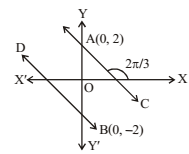Equation of the line AC passing through (0, 2) and having the slope - √3 is
y - 2 = -√3(x – 0) => √3x + y – 2 = 0
Since, another line BD is parallel to AC
∴ Slope of BD = Slope of AC = - √3
BD is passing through the point B(0, –2)
∴  Equation of BD is y + 2 = - √3 (x – 0)
=> √3x + y + 2 = 0
Thus, equation of AC and BD are √3x + y – 2 = 0 and √3x + y + 2 = 0.

15. The perpendicular from the origin to a line meets it at the point (–2, 9), find the equation of the line.

Let ON be perpendicular to AB.The point is (–2, 9)
∴ Slope of ON = (9 – 0)/(-2 – 0) = -9/2
Slope of AB which is perpendicular to ON = 2/9
(∵ Lines are perpendicular if m1m2 = –1)
Now, AB passes through (–2, 9) and has the slope 2/9 .
∴ Equation of AB, y – 9 = 2/9 (x + 2).
Or, 9y – 81 = 2x + 4 or 2x – 9y + 85 = 0.

16. The length L (in centimetres) of a copper rod is a linear function of its Celsius temperature C. In an experiment, if L = 124.942 when C = 20 and L = 125.134 when C = 110, express L in terms of C.

L is linear function of C
∴ L = a + bC
For L = 124.942, C = 20,
124.942 = a + 20 b … (i)
For L = 125.134, C = 110,
125.134 = a + 110b … (ii)
Subtracting (i) from (ii), 0.192 = 90b
∴ b = 0.192/90 = 0.00213
from (i) 124.942 = a + 20 × 0.00213
=> 124.942 = a + 0.0426
a = 124.942 – 0.0426 = 124.8994
Now L in term of C is L = a + bC
=> L = 124.8994 + 0.00213 C.

17. The owner of milk store finds that, he can sell 980 litres of milk each week at ₹14/ litre and 1220 litres of milk each week at ₹16/ litre. Assuming a linear relationship between selling price and demand, how many litres could he sell weekly at ₹17/ litre?

Let y litre milk be sold at ₹ x/litre and x, y have linear relationship.
y = a + bx i.e.
it is a straight line
Now, y 1 = 980 litre, x 1 = ₹14/ litre, y2 = 1220 litre, x 2 = ₹16/ litre
Slope of the line = (1220 – 980)/(16 – 14) = 240/2 = 120
Equation of the line, y – 980 = 120 (x – 14)
when x = 17, y = 980 + 120 (17 – 14)
= 980 + 120 × 3 = 980 + 360 = 1340
Hence, y = 1340 litre milk may be sold at ₹17 litre.

18. P(a, b) is the mid-point of a line segment between axes. Show that equation of the line is x/a + y/b = 2.

Let the line AB makes intercepts p, q on the axes.∴ A is (p, 0) and B is (0, q)
Now, P(a, b) is the mid-point of AB
∴ (p + 0)/2 = a, (0 + q)/2 = b
∴ p = 2a, q = 2b
Intercept form of the line AB is
x/p + y/q = 1 => x/2a + y/2b = 1
=> x/a + y/b = 2

19. Point R(h, k) divides a line segment between the axes in the ratio 1: 2. Find equation of the line.

Let the line AB makes intercepts a and b on the axes. The point R(h, k) divides it in the ratio 1:2∴ h = (1 × 0 + 2 × a)/(1 + 2) = 2a/3
∴ a = 3/2 h and k = (1 × b + 2 × 0)/(1 + 2) = b/3
∴ b = 3k
Intercept form of the line AB is
x/a + y/b = 1 => x/3h/2 + y/3k = 1
=> 2x/3h + y/3k = 1 => 2kx + hy = 3kh.

20. By using the concept of equation of a line, prove that the three points (3, 0), (–2, –2) and (8, 2) are collinear.